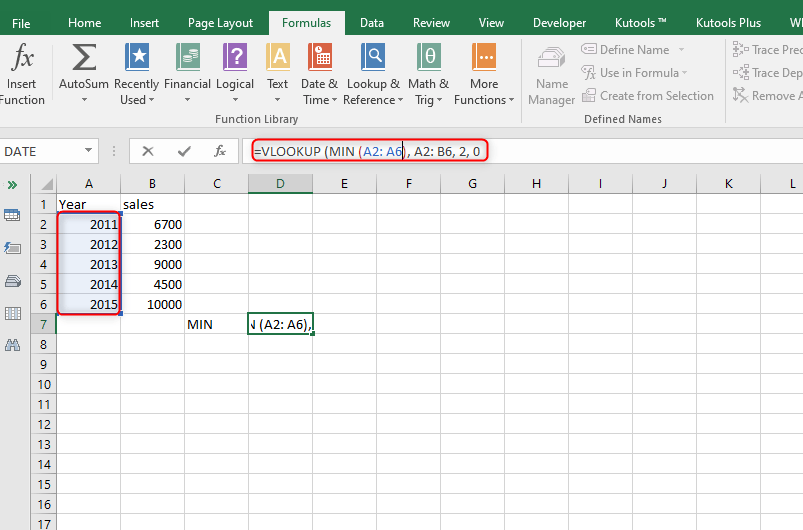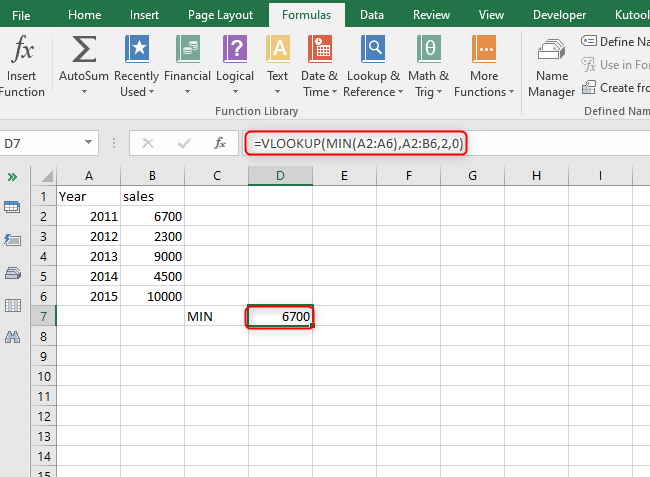# How to Vlookup to return the min value from multiple hits in Excel

Excel sheets provide several or many functions that help users perform multiple functions on a single excel sheet. These particular functions may be to do mathematical functions, graphs, filter functions, and even data validation functions.

One of the functions excel provides is the v-lookup. V-lookup is one of the excel functions that do the searching for the value you specify from one column and matches it with another value from another column. Apart from comparing values from different cells, it is also used to get the maximum and minimum values from a given data set with multiple entries.

This excel function the v-lookup, does the vertical lookup or vertical search of data as the name suggests, the v means vertical. We also have the h-lookup which does the data search horizontally. In this case, we are using the v-lookup to obtain a minimum value from multiple hits.

There are the steps used to arrive at this search and which we going to discuss in-depth together with the examples in the form of screenshots.

Step 1

Go to Microsoft Excel and open a blank excel sheet where we are going to insert the data. Ensure to have two columns one with numeral values and the other with either names or years as shown below.Step 2

The above data is not that bad for a good search. To get either the minimum or the maximum value from a certain data set there is a need for a formula since the two; min and max are mathematical functions. To do the minimum vertical lookup of data we will use the MIN function combined with the v-lookup function.The general formula for obtaining the minimum value from the excel sheet we have created will be; =VLOOKUP (MIN (A2: A7), A2: B7, 2, 0).To explain this formula, there are two columns, A and B. We will do a data search from column A and cell A2 to column B cell B7. In the minimum range for lookup, we use 0 which at times is written as false.